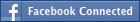• NEW! FREE Beat The GMAT QuizzesHundreds of Questions Highly Detailed Reporting Expert Explanations
• 7 CATs FREE!
If you earn 100 Forum Points

Engage in the Beat The GMAT forums to earn
100 points for $49 worth of Veritas practice GMATs FREEVERITAS PRACTICE GMAT EXAMS Earn 10 Points Per Post Earn 10 Points Per Thanks Earn 10 Points Per Upvote (n - 1) (n + 1) is divided by 24 This topic has 1 expert reply and 4 member replies GMAT/MBA Expert If n is a positive integer and r is the remainder when (n - 1) (n + 1) is divided by 24, what is the value of r? (1) n is not divisible by 2. (2) n is not divisible by 3. OA C _________________ The mind is everything. What you think you become. –Lord Buddha Sanjeev K Saxena Quantitative Instructor The Princeton Review - Manya Abroad Lucknow-226001 www.manyagroup.com Free GMAT Practice Test How can you improve your test score if you don't know your baseline score? Take a free online practice exam. Get started on achieving your dream score today! Sign up now. Senior | Next Rank: 100 PostsJoined 21 Feb 2009 Posted: 91 messages Upvotes: 2 Test Date: 15 April 2009 Target GMAT Score: 700+ Try picking numbers 1) n is not divisible by 2 n=3 (3-1)(3+1)=8 8:24=0+8 r=8 n=5 (5-1)(5+1)=24 24:24=1+0 r=0 Two different values of r, this means that the condition is insufficient 2) n is not divisible by 3 n=2 (2-1)(2+1)=3 3:24=0+3 r=3 n=5, as we know already r=0 Two different values, means condition is insufficient 1 and 2) n is not divisible by 2 and 3 n=5 r=0 n=7 (7-1)(7+1)=48 48:24=2 r=0 n=11 (11-1)(11+1)= 120 120:24=5 r=0 We can try other numbers and the result will yield to r=0, therefore answer is C. Legendary MemberJoined 22 Jul 2008 Posted: 683 messages Followed by: 2 members Upvotes: 73 (n-1), n, (n+1) are consecutive numbers. 24 = 3*(2^3) St 1: n is odd That means (n-1) and (n+1) are even 48*50 when divided by 24 the remainder is 0 26*28 when divided by 24 the remainder is not 0 Insuff St 2: n is not divisible by 3 which means either (n-1) or (n+1) is divisible by 3, because if there are 3 consecutive numbers then one of the numbers is divisible by 3. 25*27 when divided by 24 the remainder is not 0 24*26 when divided by 24 the remainder is 0 Insuff Combining 1 and 2 We know (n-1) and (n+1) are even and one of them is divisible by 3. 36*38 when divided by 24 remainder is 0 72*74 when divided by 24 remainder is 0 Suff Answer is C. GMAT/MBA Expert GMAT InstructorJoined 21 Jan 2009 Posted: 3650 messages Followed by: 81 members Upvotes: 267 GMAT Score: 760Nailya's nice; mals24's marvelous!! _________________ The mind is everything. What you think you become. –Lord Buddha Sanjeev K Saxena Quantitative Instructor The Princeton Review - Manya Abroad Lucknow-226001 www.manyagroup.com Free GMAT Practice Test How can you improve your test score if you don't know your baseline score? Take a free online practice exam. Get started on achieving your dream score today! Sign up now. Senior | Next Rank: 100 PostsJoined 12 Mar 2013 Posted: 44 messages Upvotes: 3 what is the remainder when 0/24 ? i thought it would be 0/24= quiotent 0 dividend 24 remainder 24 if n=1 (not a multiple of 2 or 3 ) (n-1)(n+1)=0 0/24 = 0 R 24 Master | Next Rank: 500 PostsJoined 01 May 2013 Posted: 149 messages Followed by: 9 members Upvotes: 54 J N wrote: what is the remainder when 0/24 ? i thought it would be 0/24= quiotent 0 dividend 24 remainder 24 0 is divisible by every number. So, when 0 is divided by any number, the remainder is 0. Apart from that, when an integer M is divided by some integer N, then the resulting operation will be of the form M = qN + r, where q and r are also integers and M = dividend, N = divisor, q = quotient, and r = remainder. And r < q < M. In this case, 0 = 0*24 + 0 As we are dividing 0 by 24, the resulting remainder cannot be greater than 0 - this should have been your clue that remainder cannot be 24. •1 Hour Free BEAT THE GMAT EXCLUSIVE Available with Beat the GMAT members only code •Magoosh Study with Magoosh GMAT prep Available with Beat the GMAT members only code •Free Veritas GMAT Class Experience Lesson 1 Live Free Available with Beat the GMAT members only code •5 Day FREE Trial Study Smarter, Not Harder Available with Beat the GMAT members only code •5-Day Free Trial 5-day free, full-access trial TTP Quant Available with Beat the GMAT members only code •Award-winning private GMAT tutoring Register now and save up to$200

Available with Beat the GMAT members only code

•Free Trial & Practice Exam
BEAT THE GMAT EXCLUSIVE

Available with Beat the GMAT members only code

•FREE GMAT Exam
Know how you'd score today for \$0

Available with Beat the GMAT members only code

•Free Practice Test & Review
How would you score if you took the GMAT

Available with Beat the GMAT members only code

•Get 300+ Practice Questions

Available with Beat the GMAT members only code

Top First Responders*

1Brent@GMATPrepNow 43 first replies
2Ian Stewart 35 first replies
3Jay@ManhattanReview 32 first replies
4GMATGuruNY 27 first replies
5Scott@TargetTestPrep 13 first replies
* Only counts replies to topics started in last 30 days
See More Top Beat The GMAT Members

Most Active Experts

1Scott@TargetTestPrep

Target Test Prep

189 posts
2Max@Math Revolution

Math Revolution

90 posts
3Brent@GMATPrepNow

GMAT Prep Now Teacher

75 posts
4GMATGuruNY

The Princeton Review Teacher

44 posts
5Ian Stewart

GMATiX Teacher

42 posts
See More Top Beat The GMAT Experts